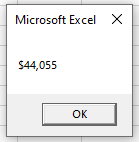# VBA Long Data Type (Dim Variable)

## Long Variable Type

The VBA Long data type is used to store very long data values (-2,147,483,648 to 2,147,483,648).  It can only store whole numbers (with no decimal places).

To declare an Long variable, you use the Dim Statement (short for Dimension):

``Dim lngA as Long``

Then, to assign a value to a variable, simply use the equal sign:

``lngA = 30000``

Putting this in a procedure looks like this:

``````Sub lngExample()
'declare the long variable
Dim lngA as Long
'populate the long variable
lngA = 30000
'show the message box
MsgBox lngA
End Sub``````

If you run the code above, the following message box will be shown.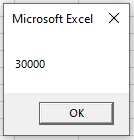### LongLong data type

The LongLong data type is only available in the 64 bit version of Microsoft Office.  If you are running a 32 bit version of Office on a 64 bit machine, this data type will not be available.  It supports numbers in the range of -9,223,372,036,854,775,808 to 9,223,372,036,854,775,807.

`` Dim lngA as LongLong``

### LongPtr data type

The LongPtr was introduced into VBA in order to support the 64 bit version of Microsoft Office.  On a 32 bit system, it is treated as a Long and on 64 bit systems it is treated as a LongLong.

``Dim lngA as LongPtr``

Note: LongPtr is not available in Excel 2007 or earlier.

### Decimal Values & Long Data Types

Long variables cannot store decimal values. If you pass a decimal number a long, the decimal number will be rounded to remove the decimal.

Therefore, if you were to run the procedure below:

``````Sub LngExampleB()
'declare the long variable
Dim lngA as Long
'populate the long variable
lngA = 3524.12
'show the message box
MsgBox lngA
End Sub
``````

The decimal value would be rounded down, returning the following message: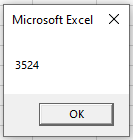However, this code below:

``````Sub LngExampleB()
'declare the long variable
Dim lngA as Long
'populate the long variable
lngA = 3524.12
'show the message box
MsgBox lngA
End Sub``````

Would return the following message box (rounding up):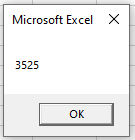### Decimal / Double Data Type

If you want to store a decimal place, you would need to declare a variable that allows for decimal places.  There are 3 data types that you can use – Single, Double or Currency.

``Dim sngPrice as Single``
``Dim dblPrice as Double``
``Dim curPrice as Currency``

The Single data type will round the decimal point slightly differently to the double and currency data type, so it is preferable to use double to single for accuracy.  A Double can have up to 12 decimal places while Currency and Single can both have up to 4 decimal places.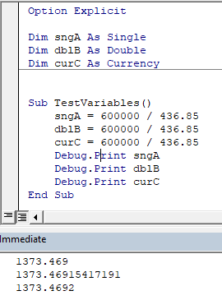## Declare Long Variable at Module or Global Level

In the previous examples, we’ve declared the Long variable within a procedure. Variables declared with a procedure can only be used within that procedure.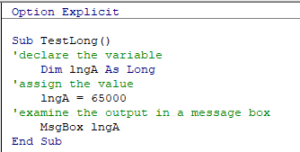Instead, you can declare Long variables at the module or global level.

### Module Level

Module level variables are declared at the top of code modules with the Dim statement.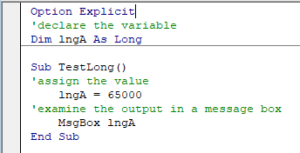These variables can be used with any procedure in that code module.

### Global Level

Global level variables are also declare at the top of code modules. However, instead of using the Dim statement, use the Public statement to indicate that the long variable is available to be used throughout your VBA Project.

``Public lngA as Long``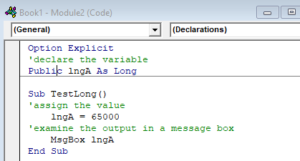If you were to declare the long variable at a module level and then try to use it in a different module, an error would occur.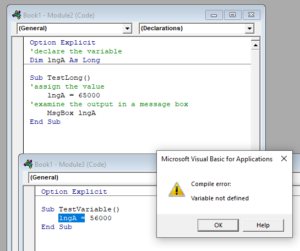However, if you had used the Public keyword to declare the long variable, the error would not occur and the procedure would run perfectly.

## Format Long Stored as String

There may be a time where you wish to format a long data type to a string – for example a date or you might want to display a currency symbol.

To achieve this, you use the Format function.

The following procedure:

``````Sub TestLongToString()
'declare the string variable
Dim strDate as string
'declare the long and populate the value
Dim lngDate as long
lngDate = 44055
'convert the long to a string formatted as a date
strDate = Format(lngDate, "dd mmmm yyyy")
'view the result
Debug.Print strDate
End Sub``````

would return the result below: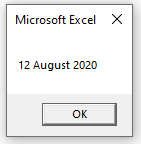and the  following procedure

``````Sub TestLongtoCurrencyString()
'declare the string variable
Dim strMoney As String
'declare the long and populate the value
Dim lngValue As Long
lngValue = 44055
'convert the long to a string with a currency symbol
strMoney = Format(lngValue, "\$#,##0")
'view the result
MsgBox strMoney
End Sub``````

would return this result: